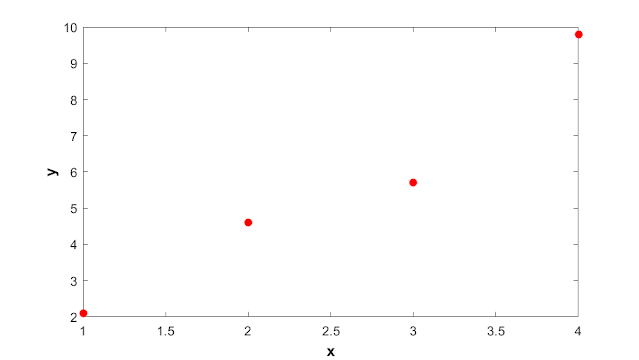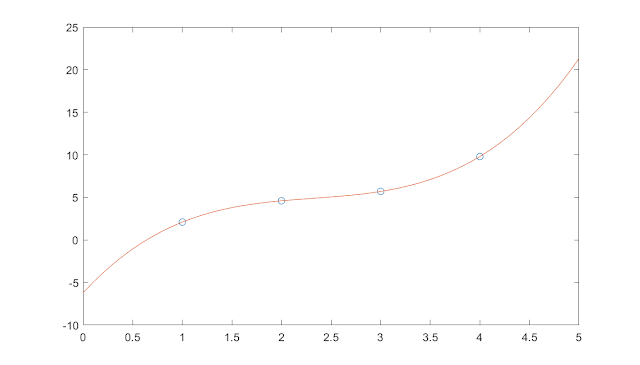## Welcome to the World of Modelling and Simulation

### What is Modelling?

This blog is all about system dynamics modelling and simulation applied in the engineering field, especially mechanical, electrical, and ...### Some Frequently used Interpolation Tools by MATLAB

Interpolation is a very essential tool in any areas of research these days. Whether you are analyzing your data or deriving equations to represent a system, interpolation plays an immense role in these works. Luckly, MATLAB has some built-in commands for basic data fitting. Not only that, you may also create your own function and use it stand alone or in combination of calling some MATLAB commands. In this article, I am going to discuss three basic techniques for basic data fitting. They are,

• Polynomial Interpolation
• Piecewise Linear Interpolation
• Cubic Spline Interpolation

Polynomial Interpolation: Polynomial interpolation is one of the most basic interpolation tools. The first step would be to learn this technique manually as it would provide us insights into the fundamentals prior to using MATLAB built-in features. The idea is that a polynomial of n degree would pass through all the (n+1) points if we apply the technique of polynomial interpolation. Let us begin with an example to strengthen the concept.
Let’s say, we have data points: (1, 2.1), (2, 4.6), (3, 5.7) and (4, 9.8). The goal is to fit a polynomial that would pass through all these points. We may consider these points as (x₁, y₁), (x2, y2), (x3, y3), and (x4, y4) where x refers to the horizontal axis and y refers to the vertical axis.Data points from the exampleNow, let's define a general polynomial equation. The following is the n-th order polynomial:If we extend the general polynomial, it looks like the following as,And, in matrix form, we may arrange these equations nicely as follows, For our example problem, we have 4 data points, which means that we will have four equations. The right side vector b is for the vertical (y-axis) data points. The left side nxn matrix is for the horizontal (x-axis) data points. But, there is a question. The horizontal data points are not in a squared matrix, specifically, it is now in 1x4 form; so, how could we represent the data as in a 4x4 matrix for our example? The following figure shows the current configuration:We can write a simple MATLAB program to make that 4x4 matrix. The following codes will generate a square matrix from a single row matrix.% Generation of a Polynomial Matrixx=[1 2 3 4]; % Sample x data pointsn = length(x); A = zeros(n,n); for i=1:n% Creating polynomial matrixfor j=1:n% nth degree polynomial passing through (n-1) pointsA(i,j) = x(i).^(j-1);endenddisp(A)After implementing the above program, we will have the following refined equations, which we can solve by Gaussian elimination to find the value of the unknown coefficients, a.Now, using the Gaussian elimination, the unknown parameters are,So, the polynomial equation that will pass through all the four points is,If we plot, the above equation fits and passes through all the points. So far, the mathematics behind polynomial interpolation has been discussed. Good news! MATLAB has a built-in polynomial interpolation feature. We can use the feature easily. You can access the built-in function from the command prompt shown as below,>> x = [1; 2; 3; 4];>> y =[2.1; 4.6; 5.7; 9.8];>> polyfit(x,y,3)ans =-6.212.667-5.100.7333'polyfit' is the built-in command in MATLAB that uses the same technique shown earlier. Piecewise Linear Interpolation: When we have more data points to fit, polynomial interpolation does generate too much oscillations in curve fitting. In this case, we may use the MATLAB built-in command 'interp1' for one dimensional piecewise linear interpolation. For our four data points example, we may write few lines in MATLAB command prompt to access this feature.>> x1 = [1;2;3;4];>> y1 =[2.1; 4.6; 5.7; 9.8];>> xx = 0:0.1:5;yy = interp1(x1,y1,xx);plot(x1,y1,'o',xx,yy)Cubic Spline Interpolation: An improvement to one dimensional piecewise linear interpolation is the cubic spline interpolation. We may use the MATLAB built-in command 'spline' for it. For our four data points example, we may write few lines in MATLAB command prompt to access this feature.>> x1 = [1;2;3;4];>> y1 =[2.1; 4.6; 5.7; 9.8];>> xx = 0:0.1:5;yy = spline(x1,y1,xx);plot(x1,y1,'o',xx,yy)#Interpolation #PiecewiseLinear #CubicSpline #Matlab #Polynomial #Blog #Blogger